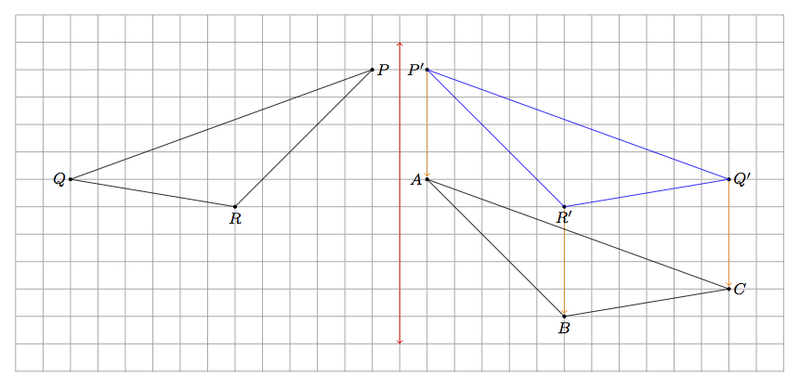# Congruent Triangles

Alignments to Content Standards: 8.G.A.2

The two triangles in the picture below are congruent:1. Give a sequence of rotations, translations, and/or reflections which take $\triangle PRQ$ to $\triangle ABC$.
2. Is it possible to show the congruence in part (a) using only translations and rotations? Explain.

## IM Commentary

This task has two goals: first to develop student understanding of rigid motions in the context of demonstrating congruence. Secondly, student knowledge of reflections is refined by considering the notion of orientation in part (b). Each time the plane is reflected about a line, this reverses the notions of ''clockwise'' and ''counterclockwise.''

In part (b) it is possible to say more. If a sequence of translations, rotations, and reflections takes triangle $PQR$ to triangle $ABC$ then the number of reflections must be odd. This is because reversing orientations an even number of times brings us back to our original notions of ''clockwise'' and ''counterclockwise.''

This task is indended for instructional purposes. Teachers should have students share their different ways of transforming triangle $PRQ$ to triangle $ABC$. The translation and reflection indicated in the solution is not the only way to solve part (a) and students should be encouraged to be creative in their use of rigid transformations.

This task includes an experimental GeoGebra worksheet, with the intent that instructors might use it to more interactively demonstrate the relevant content material. The file should be considered a draft version, and feedback on it in the comment section is highly encouraged, both in terms of suggestions for improvement and for ideas on using it effectively. The file can be run via the free online application GeoGebra, or run locally if GeoGebra has been installed on a computer.

After reading the problem, the student will click next. From here, the student has the option to choose one of two solutions. This applet will walk the student through the rigid transformations that prove the congruence. At each step, the student can proceed or go back a step. After completion of a solution, the student can go back to the solutions to view the other solution or a variation of the first. Both solutions have a second variation that is visible only after completing the solution. There are four solutions in total and clicking reset at any time will reset the problem to it's initial state.

## Attached Resources

• Geogebra File
• ## Solutions

Solution: 1

1. We may begin by translating the vertex $P$ of $\triangle PRQ$ to the corresponding vertex $A$ of $\triangle ABC$. The image of this translation is pictured below with the image of $R$ denoted $R^\prime$ and the image of $Q$ denoted $Q^\prime$ (note that the image of $P$ is $A$). This translation moves each point of $\triangle PRQ$ two boxes to the right and four boxes down.Also pictured above, along with the translated image $\triangle AR^\prime Q^\prime$ of $\triangle PQR$, is a vertical red line of reflection. Reflecting about this line will send $R^\prime$ to $R$ because $R^\prime$ is on the same horizontal line as $R$, $5$ boxes to the left of the vertical line, while $R$ is $5$ boxes to the right of the red line. Similarly this reflection will send $Q^\prime$ to $Q$ as they are on the same horizontal line with $Q^\prime$ $11$ boxes to the left and $Q$ is $11$ boxes to the right of the red line.
2. If we move from vertex $P$ to vertex $R$ to vertex $Q$ on $\triangle PRQ$ then we are moving around $\triangle PRQ$ in a clockwise direction. If we move from vertex $A$ to vertex $B$ to vertex $C$ on $\triangle ABC$, on the other hand, then we are moving in a counterclockwise direction. Both translations and rotations preserve the notions of clockwise and counterclockwise in the plane. Reflections, on the other hand, reverse these two directions. Since triangles $PRQ$ and $ABC$ have different orientations at least one reflection is needed to show this congruence.

Solution: 2. Alternate Approach to part (a)

Instead of translating first and then applying a reflection, another method would be to apply a reflection first and then a translation. Below is a line of reflection, in red, and the image, denoted $\triangle P^{\prime}Q^{\prime}R^\prime$, of $\triangle PQR$ under that reflection:The points $P,P^\prime$ lie on the same horizontal line with $P$ one unit to the left of the red line of reflection and $P^\prime$ one unit to the right. Similarly, $Q,Q^\prime$ are on the same horizontal line with $Q$ twelve units to the left and $Q^\prime$ twelve units to the right. Finally $R$ and $R^\prime$ are on the same horizontal linen with $R$ six units to the left of the line of reflection and $R^\prime$ six units to the right.

After the reflection over the red line, a translation by four boxes downward, indicated by the orange arrows, takes $\triangle P^\prime R^\prime Q^\prime$ to $\triangle ABC$